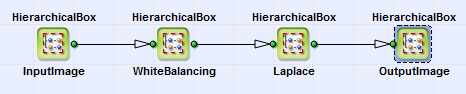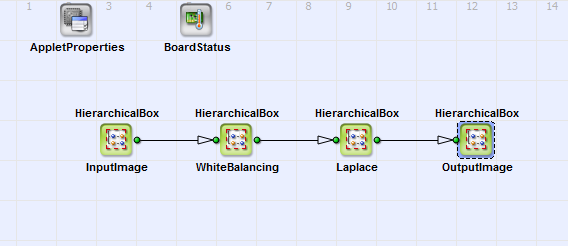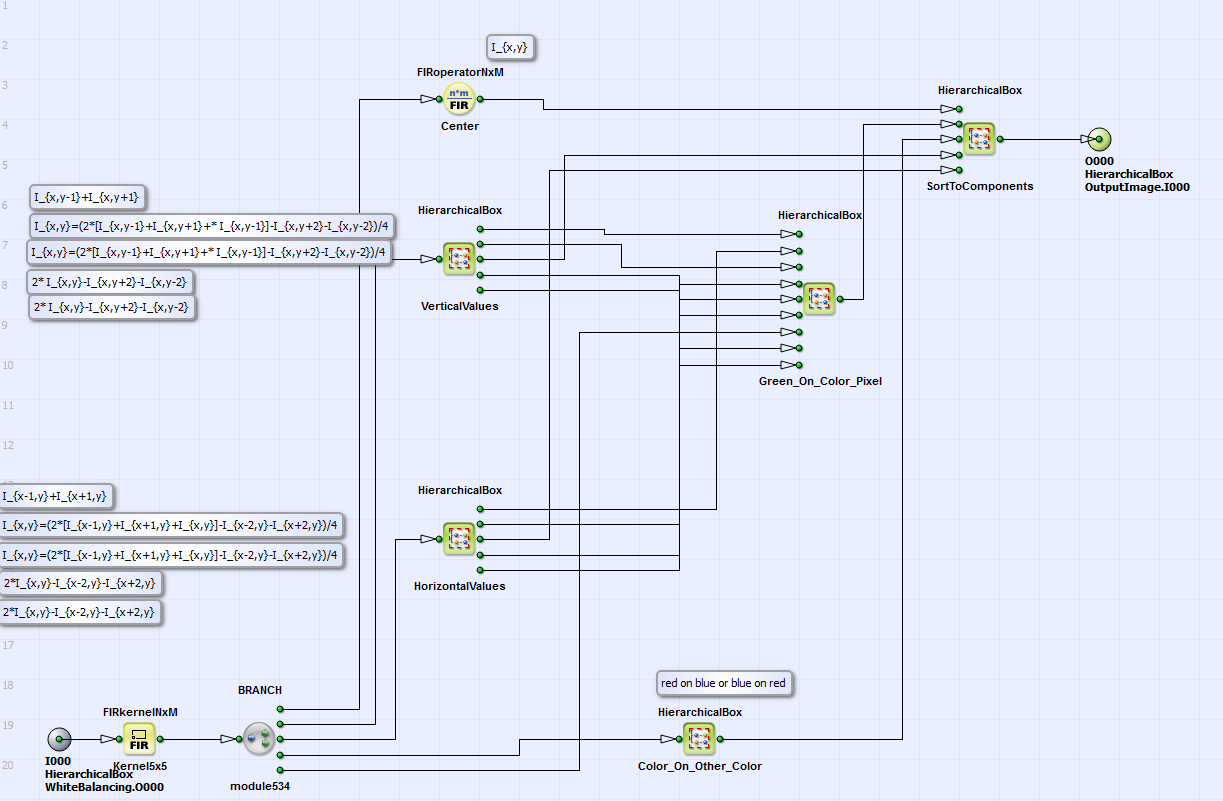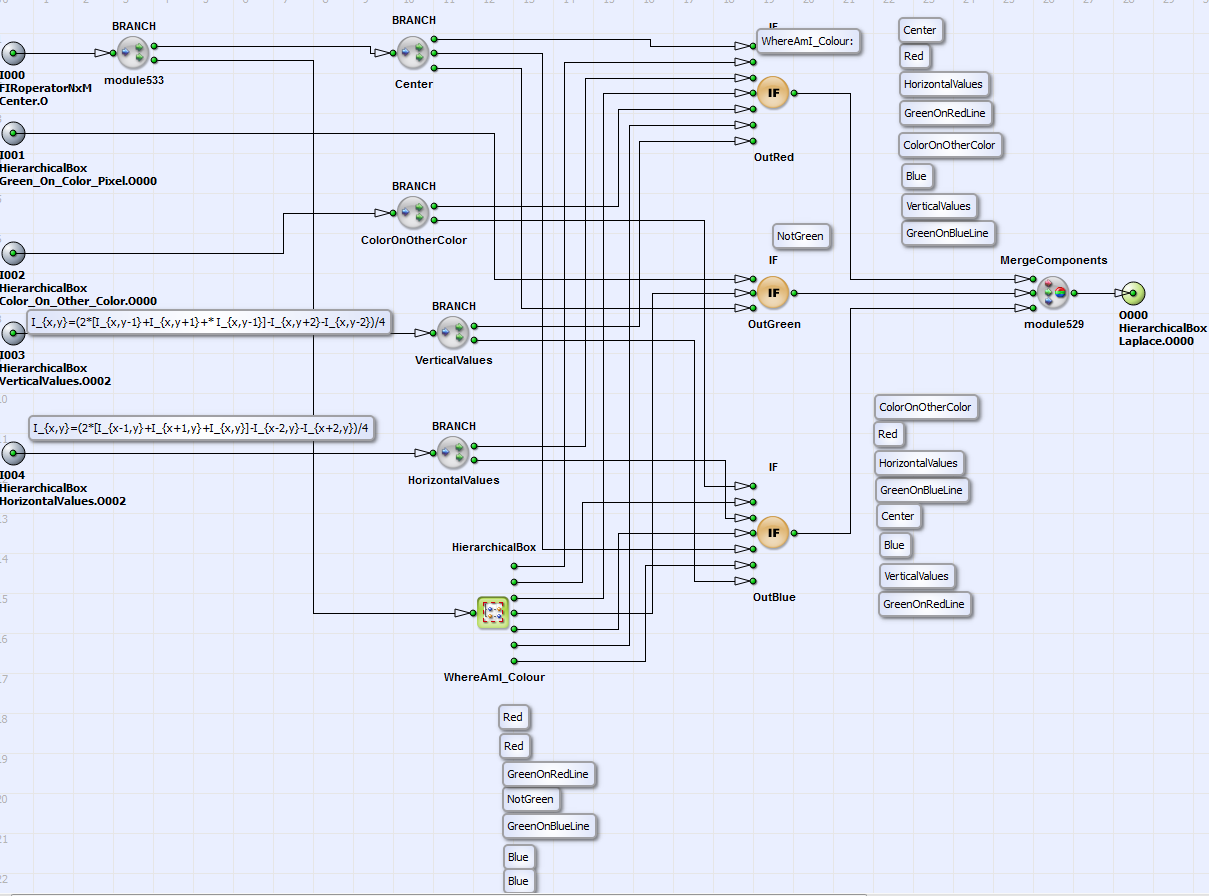#### Edge Sensitive Bayer De-Mosaicing Algorithm

Brief Description

File: \examples\Processing\Color\Bayer\LaplaceFilter_maVCL.vaDefault Platform: mE5-MA-VCL

Short Description

Laplace Edge Sensitive Bayer De-Mosaicing

In this section we give an example of an edge sensitive Bayer demosaicing algorithm, which we call "Laplace filter". In the following we first describe the interpolation algorithm and introduce shortly the implementation in VisualApplets afterwards.

##### Interpolation Algorithm

For the interpolation of the green values on red and blue pixels gradients in vertical and horizontal directions ( and ) are used:

Equation 2.

is the chrominance value (red or blue) on the pixel where the green value has to be interpolated. Green is then on the red and blue pixel positions:

Equation 3.

Here the value determines the significance of an edge. For the interpolation of the colors red and blue there exist three cases:

1. Interpolation of color on a green pixel in a line with the same color:

Equation 4.

2. Interpolation of color on a green pixel in a line with other color:

Equation 5.

3. For the interpolation of a color on a pixel with other color gradients and are used:

Equation 6.

The color (red or blue) is then:

Equation 7.

##### Implementation in VisualApplets

You can find the VisualAplets design for the Laplace filter" under \examples\Processing\Color\Bayer\LaplaceFilter_maVCL.va. In Fig. 238 you can see the basic design structure. The content of InputImage and OutputImage is equivalent to the basic acquisition examples (see e.g. 'Basic Acquisition Examples for Camera Link Cameras for marathon, LightBridge and ironman Frame Grabbers').Figure 238. Basic design structure

In Figure 239, 'Content of HierarchicalBox Laplace' the content of the HierarchicalBox Laplace is shown.Figure 239. Content of HierarchicalBox Laplace

In the HierarchicalBoxes HorizontalValues, VerticalValues, Green_On_Color_Pixel and Color_On_Other_Color the single kernel components of a kernel are used for calculation of the single equation steps of Equation 2 to Equation 7. Comment boxes give detailed information on the content of each HierarchiaclBox and the current equation step.

In Figure 240, 'Content of HierarchicalBox SortToComponents' you can see the content of the box SortToComponents. Here the calculated color values red, green and blue are assigned to the output value in dependence on the current pixel color. The color of the current pixel is determined in the HierarchicalBox WhereAmI_Colour. Here different you can choose different Bayer patterns as explained in the corresponding comment box in the example.Figure 240. Content of HierarchicalBox SortToComponents